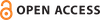BOOK REVIEW • The Dynamics of Coastal Models

First Paragraph

The basic governing equations of coastal dynamics have been known for decades, but how can these equations be formulated to improve our understanding of coastal basins? The Dynamics of Coastal Models by Clifford Hearn does a great job of explaining and illustrating fundamental coastal dynamics and equations through the use of simple analytical and numerical models. It motivates readers to further explore model physics using simple Microsoft Excel or Matlab examples from which basic ideas can be easily extracted (numerical codes are also tabulated). These examples are so simple that interested readers who have a basic math and science background can gain useful physical and mathematical insights into coastal dynamics through the simple models presented (which are never higher than two-dimensional). These models are very powerful tools, enabling clear demonstration and easy understanding of basic principles.

Citation

Y.-H. Tseng. 2009. Review of The Dynamics of Coastal Models, by C.J. Hearn. Oceanography 22(1):241–243, https://doi.org/10.5670/oceanog.2009.31.